#### Approximation algorithm framework

The lattices developed in this section were introduced in  for analyzing the kinodynamic planning problem in the rigorous approximation algorithm framework for NP-hard problems . Suppose that there are two or three independent double integrators. The analysis shows that the computed solutions are approximately optimal in the following sense. Let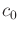and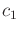be two positive constants that define a function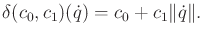(14.25)

Let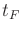denote the time at which the termination action is applied. A state trajectory is called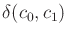-safe if for all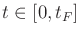, the ball of radius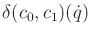that is centered at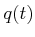does not cause collisions with obstacles in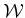. Note that the ball radius grows linearly as the speed increases. The robot can be imagined as a disk with a radius determined by speed. Let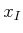,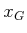,, andbe given (only a point goal region is allowed). Suppose that for a given problem, there exists a-safe state trajectory (resulting from integrating any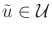) that terminates inafter time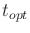. It was shown that by choosing the appropriate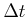(given by a formula in ), applying breadth-first search to the reachability lattice will find a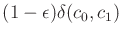-safe trajectory that takes time at most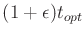, and approximately connectsto(which means that the closeness independs on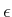). Furthermore, the running time of the algorithm is polynomial in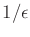and the number of primitives used to define polygonal obstacles.14.5 One of the key steps in the analysis is to show that any state trajectory can be closely tracked using only actions from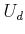and keeping them constant over. One important aspect is that it does not necessarily imply that the computed solution is close to the true optimum, as it travels through(only the execution times are close). Thus, the algorithm may give a solution from a different homotopy class from the one that contains the true optimal trajectory. The analysis was extended to the general case of open-chain robot arms in [288,441].

Steven M LaValle 2020-08-14